Download Presentation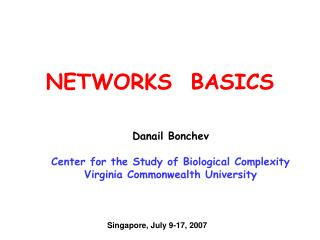NETWORKS BASICS

# NETWORKS BASICS - PowerPoint PPT Presentation

NETWORKS BASICS Danail Bonchev Center for the Study of Biological Complexity Virginia Commonwealth University Singapore, July 9-17, 2007 Recommended Literature 1. Linked: The New Science of Networks. Albert-László Barabási.I am the owner, or an agent authorized to act on behalf of the owner, of the copyrighted work described.
Download Presentation## NETWORKS BASICS

An Image/Link below is provided (as is) to download presentation

Download Policy: Content on the Website is provided to you AS IS for your information and personal use and may not be sold / licensed / shared on other websites without getting consent from its author.While downloading, if for some reason you are not able to download a presentation, the publisher may have deleted the file from their server.

- - - - - - - - - - - - - - - - - - - - - - - - - - E N D - - - - - - - - - - - - - - - - - - - - - - - - - -
Presentation Transcript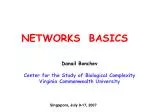### NETWORKS BASICS

Danail Bonchev

Center for the Study of Biological Complexity

Virginia Commonwealth University

Singapore, July 9-17, 2007Recommended Literature

• 1. Linked: The New Science of Networks. Albert-László Barabási.
• Perseus Publisher, 2002. ISBN: 0-738-20667-9, 304 pp., Price: \$ 15.00
• The Structure and Dynamics of Networks. Mark Newman, Albert-László
• Barabási, and Duncan J. Watts, Princeton University Press, 2006
• | \$49.50 / ISBN: 0-691-11357-2; 624 pp.
• 3. Evolution of Networks. From Biological Nets to the Internet and WWW.
• Serguei N Dorogovtsev, Jose Fernando Ferreira Mendes, and A F Ioffe
• Oxford University Press, 2003, ISBN: 0198515901, \$95.00, 344 pp.

4. An introduction to Systems Biology: Design Principles of Biological

Circuits,Uri Alon,Chapman & Hall/CRC, Taylor and Francis Group,

2006,ISBN:1584886420.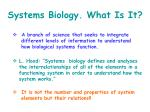Systems Biology. What Is It?

• A branch of science that seeks to integrate

different levels of information to understand

how biological systems function.

• L. Hood: “Systems biology defines and analyses

the interrelationships of all of the elements in a

functioning system in order to understand how the

system works.”

• It is not the number and properties of system

elements but their relations!!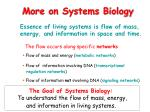The Goal of Systems Biology:

To understand the flow of mass, energy,

and information in living systems.

More on Systems Biology

Essence of living systems is flow of mass,

energy, and information in space and time.

The flow occurs along specific networks

• Flow of mass and energy (metabolic networks)
• Flow ofinformation involving DNA (transcriptional
• regulationnetworks)
• Flow of information not involving DNA (signaling networks)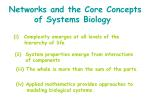Networks and the Core Concepts

of Systems Biology

• Complexity emerges at all levels of the
• hierarchy of life
• System properties emerge from interactions
• of components

(iii) The whole is more than the sum of the parts.

(iv) Applied mathematics provides approaches to

modeling biological systems.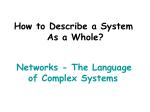How to Describe a System

As a Whole?

Networks - The Language

of Complex Systems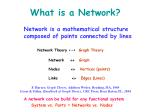What is a Network?

Network is a mathematical structure

composed of points connected by lines

Network Theory<-> Graph Theory

Network  Graph

Nodes  Vertices (points)

Links  Edges (Lines)

F. Harary, Graph Theory, Addison Wesley, Reading, MA, 1969

Gross & Yellen, Handbook of Graph Theory, CRC Press, Boca Raton, FL, 2004

A network can be build for any functional system

System vs. Parts = Networks vs. Nodes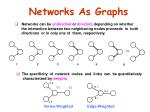1

2

3

4

5

6

2.5

12.7

7.3

3.3

5.4

8.1

2.5

Vertex-Weighted

Edge-Weighted

Networks As Graphs

• Networks can be undirected or directed, depending on whether
• the interaction between two neighboring nodes proceeds in both
• directions or in only one of them, respectively.
• The specificity of network nodes and links can be quantitatively
• characterized by weights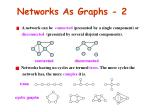A network can be connected (presented by a single component) or

• disconnected (presented by several disjoint components).

connected

disconnected

trees

cyclic graphs

Networks As Graphs - 2
• Networks having no cycles are termed trees. The more cycles the
• network has, the more complex it is.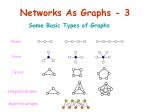Paths

Stars

Cycles

Complete Graphs

Bipartite Graphs

Networks As Graphs - 3

Some Basic Types of GraphsA. Intra-Cellular Networks

Protein interaction networks

Metabolic Networks

Signaling Networks

Gene Regulatory Networks

Composite networks

Networks of Modules, Functional Networks Disease networks

B. Inter-Cellular Networks

Neural Networks

Biological Networks

C. Organ and Tissue Networks

D. Ecological Networks

E. Evolution NetworkBio-Map

L-A Barabasi

protein-gene interactions

PROTEOME

protein-protein interactions

METABOLISM

Bio-chemical reactions

Citrate Cycle

GENOME

miRNA

regulation?

_ _ _ _ _ _ _ _ _ _ _ _ _ _ _ _ _ _ _ _ _

- -The Protein Network of Drosophila

CuraGen Corporation

Science, 2003Metabolic Networks

Source: ExPASyCleavage of Caspase

Substrates

CASP6

DISC

CASP10

Heterodimer DFF

FAS-L

FAS-R

CASP3

Death

activator

DFF45

DFF40

CASP8

Death-Inducing Signaling Complex

CASP7

Initiator

Caspases

Start DNA

Fragmentation

Executor

Caspases

Apoptosis Pathway - 1

Apoptosis is a mechanism of controlled cell death

critically important in many biological processes

Membrane

protein

D. Bonchev, L.B. Kier, C. Cheng, Lecture Serieson Computer and Computational Sciences 6, 581-591 (2006).The Longevity Gene-Protein Network (LGPN)

C. elegans

T. Witten, D. Bonchev,

in pressNetwork of Interacting Pathways (NIP)

381 organisms

A.Mazurie

D.Bonchev

G.A. Buck,

2007Cell Cycle

Cell Polarity & Structure

7

Number of protein complexes

13

111

8

61

25

40

Number of proteins

Transcription/DNA

Maintenance/Chromatin

Structure

77

19

15

Number of shared proteins

14

11

7

30

16

27

22

Intermediate

and Energy

Metabolism

187

55

740

43

221

94

33

73

83

37

103

65

11

Signaling

Membrane

Biogenesis &

Turnover

13

20

125

20

147

53

35

321

19

41

299

49

596

75

97

Protein Synthesis

and Turnover

28

692

33

419

RNA

Metabolism

260

24

172

75

12

160

Protein RNA / Transport

Functional Networks

Yeast: 1400 proteins, 232 complexes, nine functional groups of complexes

(Data A.-M. Gavin

et al. (2002) Nature

415,141-147)

D. Bonchev, Chemistry & Biodiversity 1(2004)312-326Summary

• All complex networks in nature and technology

have common features.

• They differ considerably from random networks

of the same size

• By studying network structure and dynamics, and

by using comparative network analysis, one can

get answers of important biological questions.Fundamental biological questions to answer:

(i) Which interactions and groups of interactions are likely to have

equivalent functions across species?

(ii) Based on these similarities, can we predict new functional

information about proteins and interactions that are poorly

characterized?

(iii) What do these relationships tell us about the evolution of proteins,

networks and whole species?

(iv) How to reduce the noise in biological data: Which interactions

represent true binding events?

False-positive interaction is unlikely to be reproduced across the

interaction maps of multiple species.

Fundamental Biological

(i) Which interactions and groups of interactions are likely

to have equivalent functions across species?

(ii) Based on these similarities, can we predict new functional

information about proteins and interactions that are poorly

characterized?

(iii) What do these relationships tell us about the

evolution of proteins, networks and whole species?

(iv) How to reduce the noise in biological data: Which

interactions represent true binding events?

False-positive interaction is unlikely to be reproduced

across the interaction maps of multiple species.All Complex Dynamic Networks

Have Similar Structure and

Common Properties

• Hubs
• Scale-Freeness
• Small-Worldness
• Centrality
• Robustness/FragilityQuantifying Networks

A. Graph-Theoretical (Topological) Descriptors

A1. Connectivity-based:

A2. Distance-based

B. Information-Theoretic Descriptors

B1, Compositional

B2. Structural

C. Complexity Measures

C1. Subgraph Count

C2. Overall Connectivity

C3. Walk Count

C4. Small-World Connectivity1

V = 5

E = 4

G

random node numbering

2

3

4 5

1 2 3 4 5 ai

1

0 0 1 0 0 1

0 0 1 0 0 1

1 1 0 1 0 3

0 0 1 0 1 2

0 0 0 1 0 1

1

2

3

4

5

A(G) =

1

3

2 1

node degrees

ai – node degree

Connectivity-Based Topological

Descriptors

aij= 1 (neighbors)

aij= 0 (otherwise)Connectivity Descriptors

Local (node) descriptors: vertex (node) degrees, ai

Global (Network) descriptors: total adjacency, AAverage vertex (node) degree:

Network connectedness (density)

Example

A = 1+1+3+2+1 = 8

1

<ai> = 8/5 = 1.6

Conn = 8/5.4 = 0.4 = 40%

1

3

2 1

Connectivity Descriptors-2

Average and Normalized Descriptors1

In-degree = -1

Out-degree = +1

2

3

4 5

1 2 3 4 5 ai(out)

0 0 1 0 0 +1

0 0 1 0 0 +1

1 0 0 0 0 +1

0 0 1 0 0 +1

0 0 0 1 0 +1

ai(in) -1 0 -3 -1 0

1

2

3

4

5

A(DG) =

-1,1

0,1

-3,1

-1,1 0,1

Adjacency in Directed Graphs

aij = -1 (incoming edge(arc))

aij = +1 (outgoing edge(arc))

aij = 0 (otherwise)1

2

3

4 5

6

1 2 3 4 5 di

7

0 2 1 2 3 8

2 0 1 2 3 8

1 1 0 1 2 5

2 2 1 0 1 6

3 3 2 1 0 9

1

2

3

4

5

2

3

D(G) =

4

5

d26 = ? d57 =?

Distance-Based Topological

Descriptors

Distance Matrix

Distance relation: dij = 1 for i,j - neighbors

The distance between two non-neighboring nodes is equal to the

number of edges along the shortest path that connects them.

di – node distance

(node distance degree)node distance, di

ei = Max(dij)

Node eccentricity, ei

Network distance, D(G)

Network diameter,Diam(G)

Distance Descriptors

Node descriptors:

Network descriptors:Average node distance, <di>

Average network distance, <d>

(average degree of separation, average path length)

Example

8

D = 8+8+5+6+9 = 36

<di> = 36/5 = 7.2

8

5

6 9

<d > = 36/5.4 = 1.8

Distance Descriptors-2

Average and Normalized Descriptors1

2

3 4

0,3

• Some distances in directed graphs are equal to infinity !!

-2,1

How to calculate D and <d>?

0, 2 -4, 0

Distances in Directed

Networks

• In-distances and out-distances

D(in) = D(out) = 6

<d> = 6/(4x3) = 0.5 ???

d21=? d13=?Adjusted Average Network Distance

Example:

9

9

8

Acc (G) = Nd(DG)/Nd

• Network Node Accessibility

8

7

1

0

6

8

12

G

DG

9

9

D = 52, <d(G)> = 52/(6x5) = 1.73

D = 34, <d(DG)> = 34/20 = 1.70 < D(G)??

Distances in Directed

Networks - 2

AC = 20/30 = 0.667

AD = 1.70/0.667 = 2.55 > D(G)• information content.

Information is a measure of system’s diversity

• The more complex the system, the larger its information content.

Information is a measure of system complexity

Shannon’s Information Theory
• What Is Information?

Wiener: Information is neither matter, nor energy.

• How to Measure Information?

Information is contained in any system, the elements of which can be

grouped according to one or more criteria.

References

1. Shannon, C.; Weaver, W. Mathematical Theory of Communications.University of Illinois Press: Urbana, MI, 1949.

2. Bonchev, D. Information‑Theoretic Indices for Characterization of Chemical Structures. Research Studies Press: Chichester, UK,1983.Mean Information:

, bits/element

Total Information:

, bits

Normalized Information:

Shannon’s Information Theory

Basic Equations

Finite Probability Scheme:

System of N elements and k equivalence classes with equivalence criterion α:

class number of elements probability

1N1p1

2 N2p2

………………………………………………………………...

k Nkpk

where pi = Ni / N, and Σ pi = 1.degrees

9

distances

8

eI(deg) = - 2x(2/6)log2(2/6) – 2x(1/6)log2(1/6) = 1.92 bits/node

6

8

12

9

eItot(dist) = 6log26 – 2x2log22– 2x1log1 = 11.51 bits

2

eI (deg) =11.51/(6log26) = 0.742

4

3

2

1

2

Network Information Descriptors
• Information on the system elements equivalence, eI

Vertex degree equivalence distribution: 6{3, 1, 1, 1}

eI(deg) = -(3/6)2log(3/6) – 3x(1/6)log2(1/6) = 1.79 bits/node

eItot(deg) = 6log26 – 3log23– 3x1log1 = 10.75 bits

eInorm(deg) =10.75/(6log26) = 0.693

Vertex distance equivalence distribution: 6{ 2, 2, 1, 1}

Composition distribution: 6{2,2,1,1}mI(deg) = -(4/14)log2(4/14) –(3/14)log2(3/14) –

3x(2/14)log2(2/14) – (1/14)log2(1/14) = 2.47 bits/node

degrees

mItot(deg) = 14log214 – 4log24– 3log23 -3x2log22 -1log21 = 34.55 bits

mInorm(deg) =34.55/(14log214) = 0.648

A = 14

9

distances

mI(deg) = -(12/52)log2(12/52) – 2x(9/52)log2(9/52) –

2x(8/52)log2(8/52) – (6/52)log2(6/52) = 2.55 bits/node

8

6

8

12

2

D = 52

9

4

mInorm(deg) =132.83/(52log252) = 0.448

3

2

1

2

mI(dist) = 52log252–12log212–2x9log29–2x8log28-6log26 = 132.83 bits

Network Information Descriptors - 2

Information on the system elements weight (or magnitude) , mI

weighted information descriptors (indices)

Vertex degree distribution: 14 {4, 3, 3x2, 1}

Distance magnitude distribution: 52 {12, 2x9, 2x8, 6}Network Complexity Descriptors - 1

1

1

1

1

1

1

1

3

3

3

3

2

2

2

2

1

1

1

1

1

3

Example

V = 5, E = 4

e = number of edges

1

3

e=0

0SC = 5 0OC =8

e=1

1SC = 4 1OC = 16

1

3

3

2

2

1

1

1

3

2SC = 4 2OC = 23

e=2

1

2

1

3

3

2

3

2

1

2

3SC = 3 3OC = 21

e=3

4SC=1 4OC = 8

e=4

Subgraph Count, eSC

Overall Connectivity, eOC

SC = 17 (5, 4, 4, 3, 1)

OC = 76 ( 8, 16, 23, 21, 8)Network Complexity Descriptors - 2Walk Count, WC

2

Example

1

3

4

5

l=2

l = 1

l=2

1

3

1

2

1

3

1

3

4

• The three complexity measures, SC, OC, and WC,
• can discriminate very subtle complexity features.

SC28(5,8,9,5,1) 30(5,9,10,5,1)

OC(in)111(12,28,41,25,5) 135(16,40,49,25,5)

WC 15(5,5,5) 21(5,7,9)

WC = 106 ( 8, 16, 28, 54)

• For networks use only complexity measures with e = 1, 2, and 3!!Network Complexity Descriptors - 3

Small-World Connectivity

• Network complexity increases with connectivity
• Network complexity increases with the decrease in its radius
• Can one unite the two patterns into a single complexity measure?

bi is a measure for node centrality

D. Bonchev and G. A. Buck, Quantitative Measures of Network Complexity. In: Complexity in Chemistry,

Biology and Ecology, D. Bonchev and D. H. Rouvray, Eds., Springer, New York, 2005, p. 191-235.6

3

5

7

9

8

10

SC = 29 31 54 57

OC = 190 212 482 522

WC = 178 214 300 350

B1 = 0.313 0.313 0.429 0.400

B2 = 1.6774 1.783 2.200 2.211

11

13

14

15

12

Examples of Increasing Complexity: N = 5

4

SC = 11 17 20 26

OC = 32 76 100 160

WC = 58 106 140 150

B1 = 0.2 0.222 0.250 0.333

B2 = 1.105 1.294 1.571 1.6667

SC = 61 114 119 477 973

OC = 566 1316 1396 7806 18180

WC = 337 538 638 1200 1700

A/D = 0.429 0.538 0.538 0.818 1

B2 = 2.410 2.867 2.943 4.200 5Thank You

for